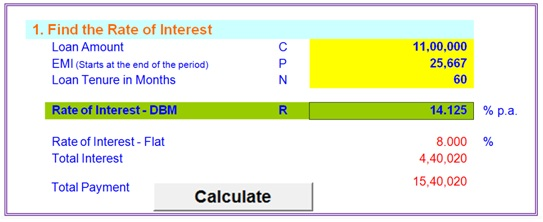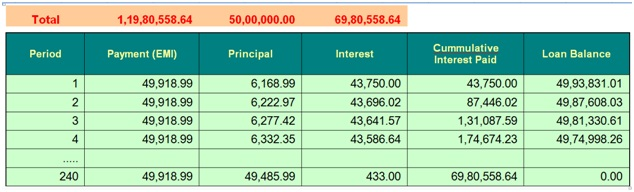# Loan Amortisation Table, Find Rate of Interest & EMI Calculator in Excel

Loan Amortisation Table, EMI Calculator, Find Rate of Interest etc. in Excel

The EMI (Equated Monthly Installment) of any loan is ascertained by the major factors viz. Loan Amount, Rate of Interest, Loan Period (Tenure).

For EMI purpose, the Interest will be calculated on Diminishing Balance Method (Monthly).

This Excel EMI Calculator, Find Rate of Interest etc will be helpful to the borrowers to cross verify the Calculations given by the Lender.

This EMI Calculator in excel has the following features;

Features :

Sheet Name “RateofInt” for 1 to 4

Please click on “Initialise (Clear)” Button to clear all the inputs.

Please click on “Sample Data” Button to prefill some Sample Data in input cells.

These Buttons are available in Row 29.

1. Find the Rate of Interest

One can find the Rate of Interest when Loan Amount, EMI and Tenure are given

This is a macro based calculation. Hence please click on “Calculation” Button after filling in the required cells2. Find the Number of Monthly Payments

One can use this when Loan Amount, EMI and Rate of Interest are given

This is formula based.

3. Calculate EMI (Monthly Payments)

One can use this when Loan Amount, Tenure and Rate of Interest are given

This is formula based.

4. Calculate EMI When Flat Rate of Interest is given

One can use this when Loan Amount, Tenure and Flat Rate of Interest are given

This is also formula based.

The parlance of using Flat rate of interest is normal in Vehicle Loan (short period loans) esp. by Private Lenders.

5. Loan Amortisation Table & EMI Calculator

Sheet Name “Loan_EMI_Table”

This Table accommodates for a maximum period for 30 years.

This will automatically generate a Report of Amortisation Table, (each one row for every month), for entire period.

This Table gives you the clear financial out flow of Total Interest Amount, Monthly Payout towards EMI, and the Loan Outstanding after each EMI.

Break up of Principal Amount and Interest Amount of each month’s EMI.

This will help accountants.

Please select in the appropriate cell w.r.t if EMI payment starts at the end of the period (default, when you don’t know) or at the beginning of the period.

Amortisation Table PreviewTags: Loan Amortisation Table, Loan Repayment Schedule, EMI Calculator, Find Rate of Interest

### More Under Finance

1. C.H. Vinay Datha says:

Thank you sir
Kindly provide the password. Mail Id – [email protected]

2. chasekh says:

thank you v.much

3. Hubert McEnroe says:

its a wonderful tool.
my email address : [email protected]

4. Pratap Shinde says:

Pl give me the excel sheet for calculation my IT for FY 2019-20.

1. Find the Rate of Interest
Rate of Interest – DBM
Not show value

6. deepa says:

Dear Sir,
send loan calculator excel with password on my mail id